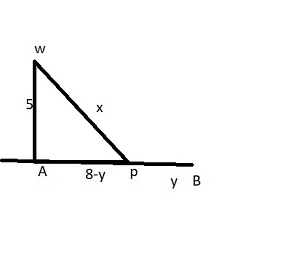# An offshore oil well is located in the ocean at a point which is 5 miles from the closest shore...

## Question:

An offshore oil well is located in the ocean at a point which is 5 miles from the closest shore point on a straight shoreline. The oil is to be piped to a shore point that is 8 miles down the shoreline by piping it on a straight line underwater from the well to some shore point and then to the storage tank 8 miles down the shoreline.

a) If the cost of laying pipe is $100,000 per mile underwater and$75,000 per miles overland, where should the pipe be located to minimize the cost of laying the pipe?

b) What is the minimizing cost( to the nearest dollar)?

## Cost of minimization

It is one of the tool used to find what number of labor N finally produces the resultant output at the minimum cost.

It can also be defined as the most effective method of sending goods while maintaining quality upto a certain level.

## Answer and Explanation:{eq}Cost\ of\ pipe=cost\ of\ under\ water\ +cost\ of\ load\\ =100000x+75000y\\ Now,\ substitue\ the\ value\ of x\\\\ C(y)=100000(\sqrt{25+(8-y)^2})+75000y\\ Now\ differentiating\ it\ with\ respect\ to\ y\\ C'(y)=100000(\frac{1}{2}(25+(8-y)^2)^\frac{-1}{2})+75000\\ =-100000(8-y)(25+(8-y^2))^{\frac{-1}{2}}+75000\\ Now,\ for\ critical\ points\\ C'(y)=0\\ \therefore y=2.33\\ to\ place\ is\ 2.33m\ to\ left\ of\ B.\\ Now,\\ x=\sqrt{25+(8-2.33)^2}=7.58\\ \therefore Cost\ minimum\ =100000*7.58+75000*2.33\\ =9.32750 {/eq}